# Class 10 RD Sharma Solutions – Chapter 3 Pair of Linear Equations in Two Variables – Exercise 3.2 | Set 1

• Last Updated : 16 May, 2021

### 2x + 5y = 12

Solution:

Given that, 2x + 5y = 12 and x + y = 3

Attention reader! All those who say programming isn't for kids, just haven't met the right mentors yet. Join the  Demo Class for First Step to Coding Coursespecifically designed for students of class 8 to 12.

The students will get to learn more about the world of programming in these free classes which will definitely help them in making a wise career choice in the future.

We have,

x + y = 3,

When y = 0, we get x = 3

When x = 0, we get y = 3

So, the following table giving points on the line x + y = 3:

Now, 2 + 5y = 12

y = (12 – 2x)/5

When x = 1, we have

y = (12 – 2)/5 = 4

When x = -4, we have

y = (12 – 2(-4))/5 = 4

So, the following table giving points on the line 2x + 5y = 12:

So, the graph of the equation x + y = 3 and 2x + 5y = 12 isFrom the graph we conclude that the two lines intersect at a point P (1, 2).

Hence x = 1 and y = 2 are required point.

### 2x + 3y = 10

Solution :

Given that,, x – 2y = 5 and 2x + 3y = 10

Now, x – 2y = 5

x = 5 + 2y

When y = 0, we get x = 5

When y = -2, we get x = 1

So, the following table giving points on the line x – 2y = 5

Now,  2x + 3y = 10

x = (10 – 3y)/2

When y = 0, we get x = 5

When y = 2, we get x = 2

So, the following table giving points on the line 2x + 3y = 10

So, the graph of the equation x – 2y = 5 and 2x + 3y = 10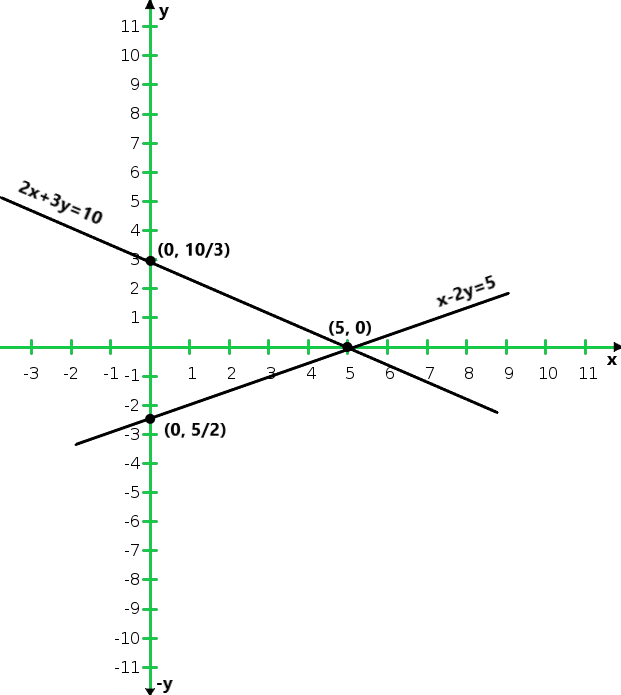From the graph we conclude that the two lines intersect at a point P (5, 0)

Hence x = 5 and y = 0 are required point.

### 2x – 3y + 8 = 0

Solution:

Given that, 3x + y + 1 = 0 and 2x – 3y + 8 = 0

Now 3x + y + 1 = 0

y = -1 – 3x

When x = 0, we get x = -1

When y = -1, we get x = 2

So, the following table giving points on the line x – 2y = 5

Now,  2x – 3y + 8 = 0

x = (3y – 8)/2

When y = 0, we get x = -4

When y = 2, we get x = 1

So, the following table giving points on the line 2x + 3y = 10

So, the graph of the equation 3x + y + 1 = 0 and 2x – 3y + 8 = 0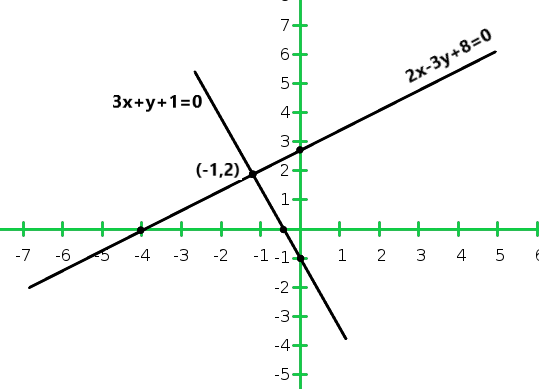From the graph we conclude that the two lines intersect at a point P (-1, 2)

Hence x = -1 and y = 2 are required points.

### 2x – 3y – 7 = 0

Solution:

Given that,, 2x + y – 3 = 0 and 2x – 3y – 7 = 0

Now 2x + y – 3 = 0,

y = 3 – 2x

When x = 0, we get x = 3

When x = 1, we get x = 1

So, the following table giving points on the line 2x + y – 3 = 0

Now,   2x – 3y – 7 = 0

When x = 0, we get y = 1

When x = 2, we get y = -1

So, the following table giving points on the line 2x + 3y = 10

So, the graph of the equation 2x + y – 3 = 0 and 2x – 3y – 7 = 0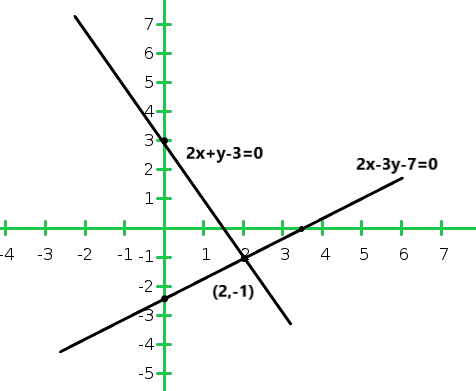From the graph we conclude that the two lines intersect at a point P (2,-1)

Hence x = 2 and y = -1 are required points.

### x – y = 2

Solution:

Given that, x + y = 6 and x – y = 2

Now x + y = 6

y = 6 – x

When x = 2, we get y = 4

When x = 3, we get y = 3

So, the following table giving points on the line x + y = 6

Now ,  x – y = 2

y = x – 2

When x = 0, we get y = – 2

When x = 2, we get y = 0

So, the following table giving points on the line x – y = 2

So, the graph of the equation x + y = 6 and x – y = 2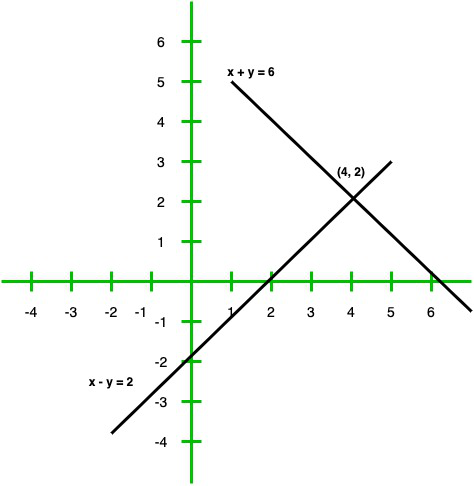From the graph we conclude that the two lines intersect at a point P (4, 2)

Hence x = 4 and y = 2 are required points.

### 3x – 6y = 0

Solution:

Given that,   x – 2y = 6 and 3x – 6y = 0

Now x – 2y = 6

x = 6 + 2y

When y = -2, we get x = 2

When y = -3, we get x = 0

So, the following table giving points on the line x – 2y = 6

Now,  3x – 6y = 0

= x = 2y

When y = 0, we get y = 0

When y = -1, we get x = 2

So, the following table giving points on the line 3x – 6y = 0

So, the graph of the equation x – 2y = 6 and 3x – 6y = 0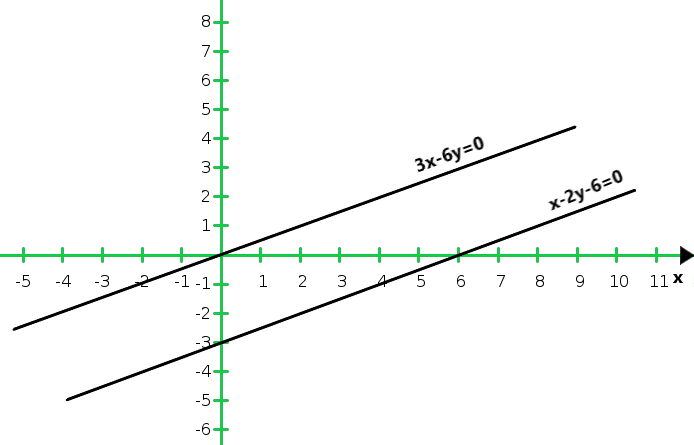From the graph we conclude that the two lines are parallel to each other so, the two lines have no common point.

Hence the given system has no solutions.

### 2x – 3y = 3

Solution:

Given that,   x + y = 4 and 2x – 3y = 3

Now x + y = 4

x = 4 – y

When y = 0, we get x = 4

When y = 2, we get x = 2

So, the following table giving points on the line x + y = 4

Now ,  2x – 3y = 3

x = (3y + 3)/2

When y = 1, we get x = 3

When y = -1, we get x = 0

So, the following table giving points on the line 2x – 3y = 3

So, the graph of the equation x + y = 4 and 2x – 3y = 3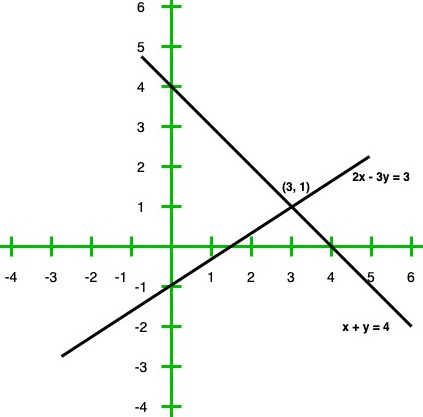From the graph we conclude that the two lines intersect at a point P(3, 1)

Hence x = 3 and y = 1 are required points.

### x – y + 3 = 0

Solution:

Given that, 2x + 3y = 4 and x – y + 3 = 0

Now 2x + 3y = 4

x = (4 – 3y)/2

When y = 0, we get x = 2

When y = 2, we get x = – 1

So, the following table giving points on the line x + y = 4

Now,  x – y + 3 = 0

x = y – 3

When y = 3, we get x = 0

When y = 4, we get x = 1

So, the following table giving points on the line x – y + 3 = 0

So, the graph of the equation 2x + 3y = 4 and x – y + 3 = 0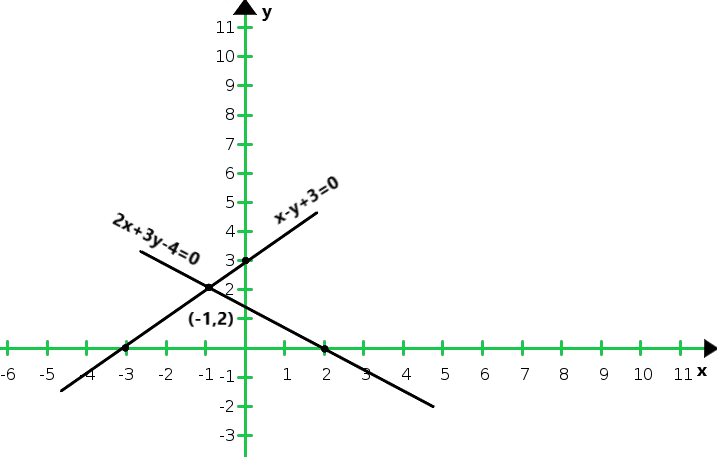From the graph we conclude that the two lines intersect at (-1, 2)

Hence x = -1 and y = 2 are required points.

### 3x – 2y + 12 = 0

Solution:

Given that, 2x – 3y + 13 = 0 and 3x – 2y + 12 = 0

Now,  2x – 3y + 13 = 0

x = (3y – 13)/2

When y = 1, we get x = – 5

When y = 3, we get x = – 2

So, the following table giving points on the line 2x – 3y + 13 = 0

Now,  3x – 2y + 12 = 0

x = (2y – 12)/3

When y = 0, we get x = -14

When y = 3, we get x = -2

So, the following table giving points on the line x – y + 3 = 0

So, the graph of the equation 2x – 3y + 14 = 0 and 3x – 2y + 12 = 0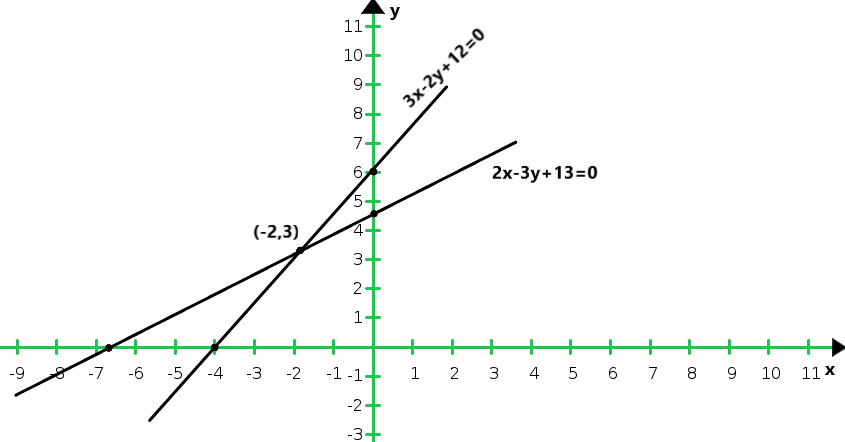From the graph we conclude that the two lines intersect at (- 2, 3)

Hence x = – 2 and y = 3 are required points.

### 3x – 2y – 12 = 0

Solution:

Given that, 2x + 3y + 5 = 0 and 3x – 2y – 12 = 0

Now,  2x + 3y + 5 = 0,

x = (-3y – 5)/2

When y = 1, we get x = – 4

When y = -1, we get x = -1

So, the following table giving points on the line 2x + 3y + 5 = 0

Now,  3x – 2y – 12 = 0,

x = (2y + 12)/3

When y = 0, we get x = 4

When y = 3, we get x = 6

So, the following table giving points on the line 3x – 2y – 12 = 0

So, the graph of the equation 2x + 3y + 5 = 0 and 3x – 2y – 12 = 0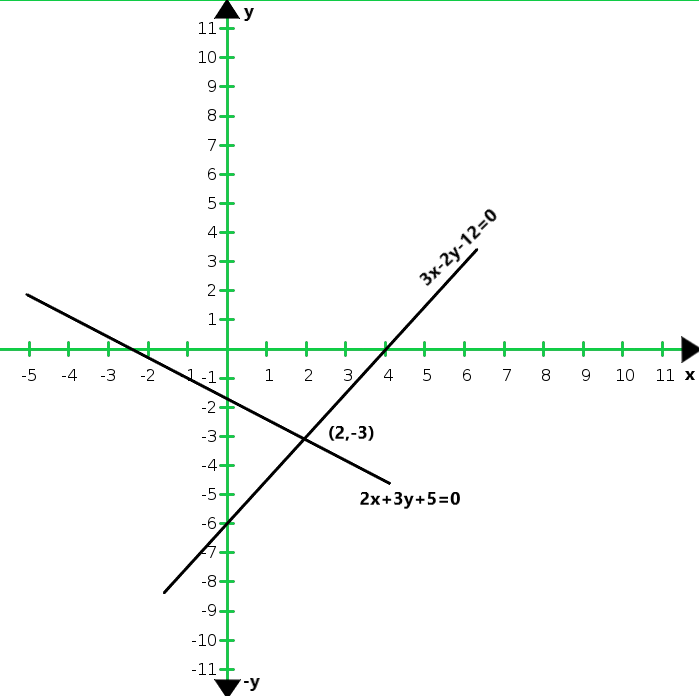From the graph we conclude that the two lines intersect at (2, 3).

Hence x = 2 and y = 3 are required points.

### 4x + 6y = 12

Solution:

Given that, 2x + 3y = 6 and 4x + 6y = 12

Now,  2x + 3y = 5

x = (6-3y)/2

When y = 0, we get x = 3

When y = 2, we get x = 0

So, the following table giving points on the line 2x + 3y = 6

Now,   4x + 6y = 12

x = (12 – 6y)/4

When y = 0, we get x = 3

When y = 2, we get x = 0

So, the following table giving points on the line 4x + 6y = 12

So, the graph of the equation 2x + 3y = 6 and 4x + 6y = 12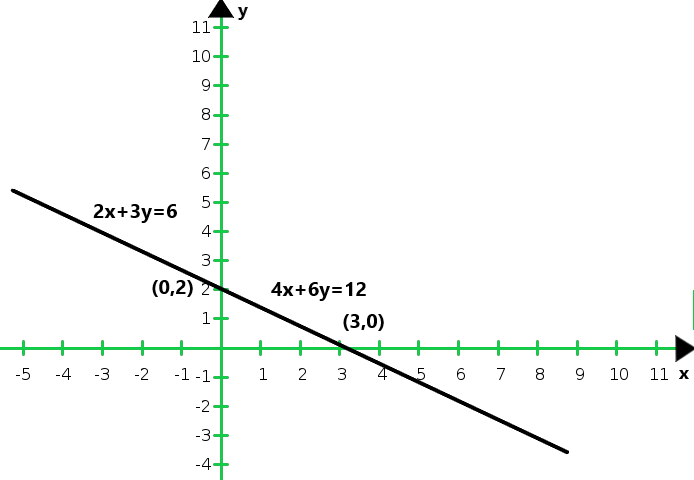From the graph we conclude that the two equations are coincident.

Hence, the system of equations has infinitely many solutions.

### 3x – 6y = 15

Solution:

Given that, x – 2y = 5 and 3x – 6y = 15

Now, x – 2y = 5

x = 2y + 5

When y = -1, we get x = 3

When y = 0, we get x = 5

So, the following table giving points on the line x – 2y = 5

Now, 3x – 6y = 15

x = (15 + 6y)/3

When y = -2, we get x = 1

When y = -3, we get x = -1

So, the following table giving points on the line 3x – 6y = 15

So, the graph of the equations x – 2y = 5 and 3x – 6y = 15: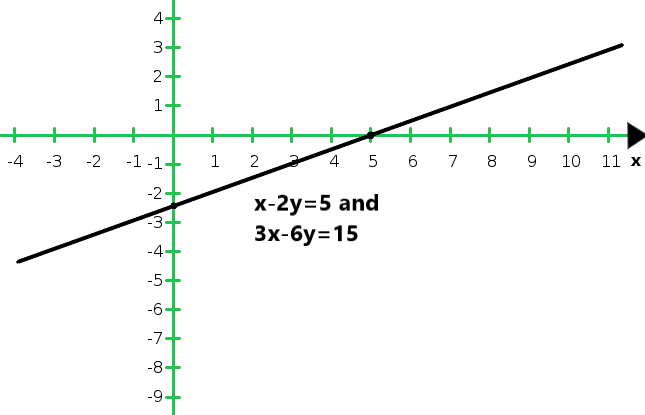From the graph we conclude that the two equations are coincident.

Hence, the system of equations has infinitely many solutions.

### 6x + 2y = 16

Solution:

Given that, 3x + y = 8 and 6x + 2y = 16

Now, x – 2y = 5

= y = 8 – 3x

When x = 2, we get y = 2

When x = 3, we get y = -1

So, the following table giving points on the line 3x + y = 8

Now, 6x + 2y = 16

y = (16 – 6x)/2

When x = 1, we get y = 5

When x = 3, we get y = -1

So, the following table giving points on the line 6x + 2y = 16

So, the graph of the given equations 3x + y = 8 and 6x + 2y = 16: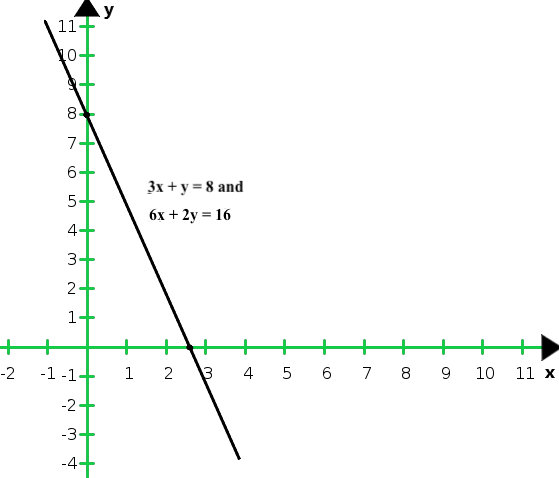From the graph we conclude that the graphs of the two equations are coincident

Hence, the system of equations has infinitely many solutions.

My Personal Notes arrow_drop_up